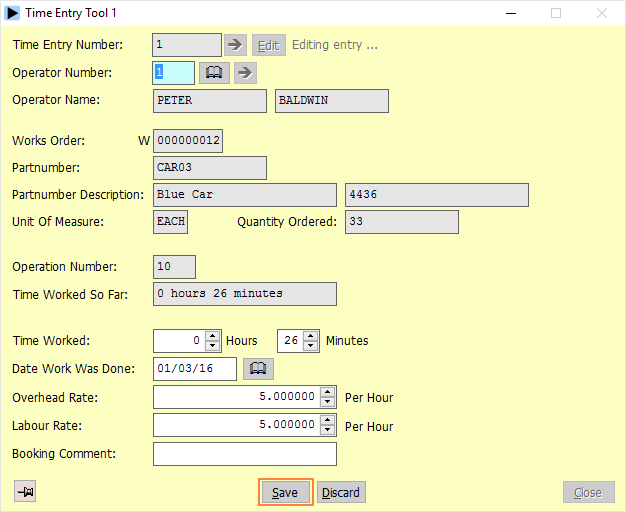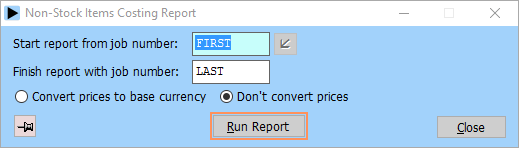As work is performed on a works order the hours worked are recorded on the route card (or a similar document) on an operation-by-operation basis.  These times are entered into Herschel using the record time worked option:

The following is recorded for each time entry:

 operator number The number of the operator who performed the work. works order number The works order number. operation number The operation number the time has been worked on. time worked The time worked in minutes and hours. date The date the work was done. overhead rate The overhead rate per hour for the work. labour rate The labour rate per hour for the work. booking comment A booking comment.

Any errors can be corrected using the time worked tool:Herschel can produce either a job cost summary or job cost details report.  The costing logic is the same for both; the detailed report gives a fuller picture of how the costs are built up:All costs can be based on standard, average or latest costs.   Tooling items can be omitted if required.  The logic used to calculate the cost of a job is as follows:

 Overhead Cost Sum of hours worked x overhead rate for all time entries Labour Cost Sum of hours worked x labour rate for all time entries Material Cost Sum of quantity issued x cost for all kit lines Total Cost Overhead Cost + Labour Cost + Material Cost

The reports highlight any jobs where the actual cost exceeds that expected, and the summary report will, if required, only list those where actual cost is higher than expected.

The non-stock items report produces a list of non-stock items for a given range of job numbers.  This can be useful when costing a job that includes purchased non-stock items:The WIP valuation reports allow an accurate valuation of work in progress on the shop floor to be made for a range of works orders.  The cost so far of each job is calculated, and the expected cost of any of the order already received into stock is deducted. This gives the total value of the part of the order still out on the shopfloor.  This figure is then totalled for all selected works orders to give a total WIP valuation.  The two reports offer the same information in different formats - pick the one which keeps your accountant happy:The time worked report totals the number of items produced and hours worked for a given range of operators, dates and orders:The transfer costs to stock file option allows the calculated job costs for a range of works orders to be passed back into the stock file, where the standard, average or latest costs can be updated: